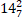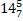# Quiz Discussion

The average age of a class is 15.8 years. The average age of the boys in the class is 16.4 years while that of the girls is 15.4 years. What is the ratio of boys to girls in the class?

Course Name: Quantitative Aptitude

• 1]

1:2

• 2]

2:3

• 3]

3:4

• 4]

3:5

##### Solution
No Solution Present Yet

#### Top 5 Similar Quiz - Based On AI&ML

Quiz Recommendation System API Link - https://fresherbell-quiz-api.herokuapp.com/fresherbell_quiz_api

# Quiz
1
Discuss

The average weight of a class of 24 students is 35 kg. If the weight of the teacher be included, the average rises by 400 g. The weight of the teacher is -

• 1] 45 kg
• 2] 50 kg
• 3] 53 kg
• 4] 55 kg
##### Solution
2
Discuss

The average of five different positive numbers is 25. x is the decrease in the average when the smallest number among them is replaced by 0. What can be said about x?

• 1] x is less than 5
• 2] x is greater than 5
• 3] x is equal to 5
• 4] Cannot be determined
##### Solution
3
Discuss

There were 35 students in a hostel. Due to the admission of 7 new students the expenses of the mess were increased by Rs.42 per day while the average expenditure per head diminished by Re 1. What was the original expenditure of the mess?

• 1] Rs. 450
• 2] Rs. 320
• 3] Rs. 550
• 4] Rs. 420
##### Solution
4
Discuss

The average age of four boys A, B, C and D is 5 years and the average age A, B, D, E is 6 years. C is 8 years old. The age of E is (in years) :

• 1] 12
• 2] 13
• 3] 14
• 4] 15
##### Solution
5
Discuss

Total expenses of a boarding house are partly fixed and partly varying linearly with the number of boarders. The average expense per boarder is Rs. 700 when there are 25 boarders and Rs. 600 when there are 50 boarders. What is the average expense per boarder when there are 100 boarders?

• 1] Rs. 540
• 2] Rs. 550
• 3] Rs. 570
• 4] Rs. 580
##### Solution
6
Discuss

The average monthly salary of 660 workers in a factory is Rs. 380. The average monthly salary of officers is Rs. 2100 and the average monthly salary of the other workers is Rs. 340. Find the number of other workers.

• 1] 645
• 2] 650
• 3] 640
• 4] 642
##### Solution
7
Discuss

The average score of a class of boys and girls in an examination is A. The ratio of boys and girls in the class is 3 : 1. If the average score of the boys is A + 1, the average score of the girls is-

• 1] A - 1
• 2] A - 3
• 3] A + 1
• 4] A + 3
##### Solution
8
Discuss

The average of the largest and smallest 3 digits numbers formed by 0, 2 and 4 would be :

• 1] 312
• 2] 222
• 3] 213
• 4] 303
##### Solution
9
Discuss

A man started his journey from Lucknow to Kolkata, which is 200 km, at the speed of 40 kmph then he went to Banglore which is 300 km, at the speed of 20 kmph. Further he went to Ahmedabad which is 500 km, at the speed of 10 kmph. The average speed of the man is :

• 1]kmph

• 2]Kmph

• 3]

15.6 kmph

• 4]

16.1 kmph

##### Solution
10
Discuss

Out of 20 boys, 6 are each of 1 m 15 cm height, 8 are of 1 m 10 cm and rest of 1 m 12 cm. The average height of all of them is :

• 1] 1 m 12.1 cm
• 2] 1 m 21.1 cm
• 3] 1 m 21 cm
• 4] 1 m 12 cm
# Quiz